# Universality classes

Universality classes are groups of models that have the same set of critical exponents

 dimension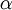$\alpha$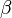$\beta$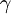$\gamma$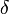$\delta$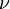$\nu$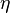$\eta$ class 3-state Potts Ashkin-Teller Chiral Directed percolation 2 0 1/8 7/4 1 1/4 2D Ising 3 0.1096(5) 0.32653(10) 1.2373(2) 4.7893(8) 0.63012(16) 0.03639(15) 3D Ising Local linear interface any 0 1/2 1 3 1/2 0 Mean-field Molecular beam epitaxy Random-field 3 −0.0146(8) 0.3485(2) 1.3177(5) 4.780(2) 0.67155(27) 0.0380(4) XY

where

•$\alpha$ is known as the heat capacity exponent
•$\beta$ is known as the magnetic order parameter exponent
•$\gamma$ is known as the susceptibility exponent
•$\delta$ is known as the equation of state exponent
•$\nu$ is known as the correlation length exponent
•$\eta$ is known as the anomalous dimension in the critical correlation function.

# Derivations

## Ising

The Hamiltonian of the Ising model is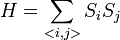$H=\sum_{}S_i S_j$

where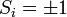$S_i=\pm 1$ and the summation runs over the lattice sites.

The order parameter is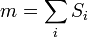$m=\sum_i S_i$

In two dimensions, Onsager obtained the exact solution in the absence of a external field, and the critical exponents are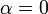$\alpha=0$

(In fact, the specific heat diverges logarithmically with the critical temperature)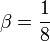$\beta=\frac{1}{8}$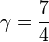$\gamma=\frac{7}{4}$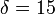$\delta=15$

along with :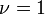$\nu=1$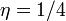$\eta = 1/4$

In three dimensions, the critical exponents are not known exactly. However, Monte Carlo simulations and Renormalisation group analysis provide accurate estimates :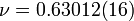$\nu=0.63012(16)$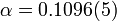$\alpha=0.1096(5)$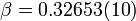$\beta= 0.32653(10)$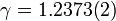$\gamma=1.2373(2)$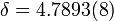$\delta=4.7893(8)$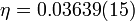$\eta =0.03639(15)$

with a critical temperature of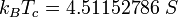$k_BT_c = 4.51152786~S$. In four and higher dimensions, the critical exponents are mean-field with logarithmic corrections.

## Mean-field

The critical exponents of are derived as follows :

#### Heat capacity exponent:$\alpha$

(final result:$\alpha=0$)

#### Magnetic order parameter exponent:$\beta$

(final result: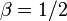$\beta=1/2$)

#### Susceptibility exponent:$\gamma$

(final result: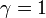$\gamma=1$)

#### Equation of state exponent:$\delta$

(final result: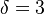$\delta=3$)

#### Correlation length exponent:$\nu$

(final result: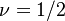$\nu=1/2$)

#### Correlation function exponent:$\eta$

(final result: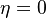$\eta=0$)

## XY

For the three dimensional XY model one has the following critical exponents: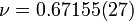$\nu=0.67155(27)$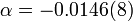$\alpha = -0.0146(8)$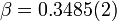$\beta= 0.3485(2)$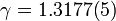$\gamma=1.3177(5)$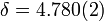$\delta=4.780(2)$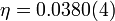$\eta =0.0380(4)$